# What are bits, bytes, and other units of measure for digital information?

A bit is a binary digit, the smallest increment of data on a computer. A bit can hold only one of two values: 0 or 1, corresponding to the electrical values of off or on, respectively.

Because bits are so small, you rarely work with information one bit at a time. Bits are usually assembled into a group of eight to form a byte. A byte contains enough information to store a single ASCII character, like "h".

An expression to calculate how many different numbers can be represented by an 8-bit binary number:

2 x 2 x 2 x 2 x 2 x 2 x 2 x 2

or 28

A kilobyte (KB) is 1,024 bytes, not one thousand bytes as might be expected, because computers use binary (base two) math, instead of a decimal (base ten) system.

Computer storage and memory is often measured in megabytes (MB) and gigabytes (GB). A medium-sized novel contains about 1 MB of information. 1 MB is 1,024 kilobytes, or 1,048,576 (1024x1024) bytes, not one million bytes.

Similarly, one 1 GB is 1,024 MB, or 1,073,741,824 (1024x1024x1024) bytes. A terabyte (TB) is 1,024 GB; 1 TB is about the same amount of information as all of the books in a large library, or roughly 1,610 CDs worth of data. A petabyte (PB) is 1,024 TB. 1 PB of data, if written on DVDs, would create roughly 223,100 DVDs, i.e., a stack about 878 feet tall, or a stack of CDs a mile high. Indiana University is now building storage systems capable of holding petabytes of data. An exabyte (EB) is 1,024 PB. A zettabyte (ZB) is 1,024 EB. Finally, a yottabyte (YB) is 1,024 ZB.

Many hard drive manufacturers use a decimal number system to define amounts of storage space. As a result, 1 MB is defined as one million bytes, 1 GB is defined as one billion bytes, and so on. Since your computer uses a binary system as mentioned above, you may notice a discrepancy between your hard drive's published capacity and the capacity acknowledged by your computer. For example, a hard drive that is said to contain 10 GB of storage space using a decimal system is actually capable of storing 10,000,000,000 bytes. However, in a binary system, 10 GB is 10,737,418,240 bytes. As a result, instead of acknowledging 10 GB, your computer will acknowledge 9.31 GB. This is not a malfunction but a matter of different definitions.

We count in base 10 by powers of 10:

```  101 = 10
102 = 10*10 =  100
103 = 10*10*10 = 1,000
106 = 1,000,000```

Computers count by base 2:

```  21 = 2
22 = 2*2 = 4
23 = 2*2*2 = 8
210 = 1,024
220 = 1,048,576```

So in computer jargon, the following units are used:

Unit Equivalent
1 kilobyte (KB) 1,024 bytes
1 megabyte (MB) 1,048,576 bytes
1 gigabyte (GB) 1,073,741,824 bytes
1 terabyte (TB) 1,099,511,627,776 bytes
1 petabyte (PB) 1,125,899,906,842,624 bytes

Bytes in a terabyte.....

1024⁴ = 1024×1024×1024×1024 = 1099511627776

Note: The names and abbreviations for numbers of bytes are easily confused with the notations for bits. The abbreviations for numbers of bits use a lower-case "b" instead of an upper-case "B". Since one byte is made up of eight bits, this difference can be significant. For example, if a broadband Internet connection is advertised with a download speed of 3.0 Mbps, its speed is 3.0 megabits per second, or 0.375 megabytes per second (which would be abbreviated as 0.375 MBps). Bits and bit rates (bits over time, as in bits per second [bps]) are most commonly used to describe connection speeds, so pay particular attention when comparing Internet connection providers and services.

Data Storage
One extended-ASCII character in a text file (eg 'A') 1 byte
The word 'Monday' in a document 6 bytes
A plain-text email 2 KB
64 pixel x 64 pixel GIF 12 KB
Hi-res 2000 x 2000 pixel RAW photo 11.4 MB
Three minute MP3 audio file 3 MB
One minute uncompressed WAV audio file 15 MB
One hour film compressed as MPEG4 4 GB

Task: Create a powerpoint including revision information on bits & bytes. Include expressions to show how many bits are in .......

• A byte.
• A nibble.
• For the following write expressions to show both how many bits and bytes are in each unit. Make sure you are clear on the difference!
• A kilobyte.
• A gigabyte.
• A terabyte.
• For all the byte classifications listed in the text above (up to Yottabyte) Create images to represent their size and capacity (i.e.1 PB  = 100 dvd's). This will help you to revise as you will have a viasual image to remind you of the storage capacity.

Next Task: Past the past paper questions below into your notes then use the internet to research model answers.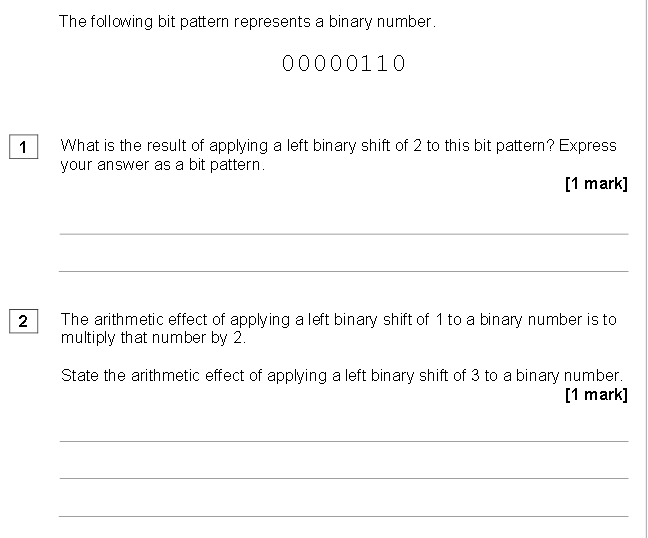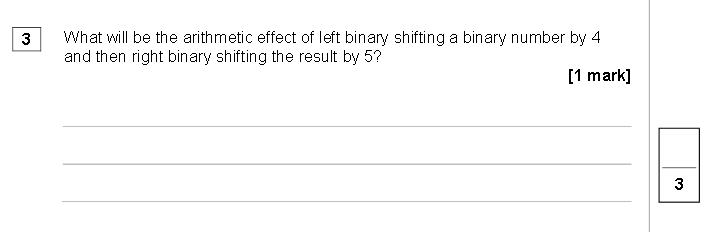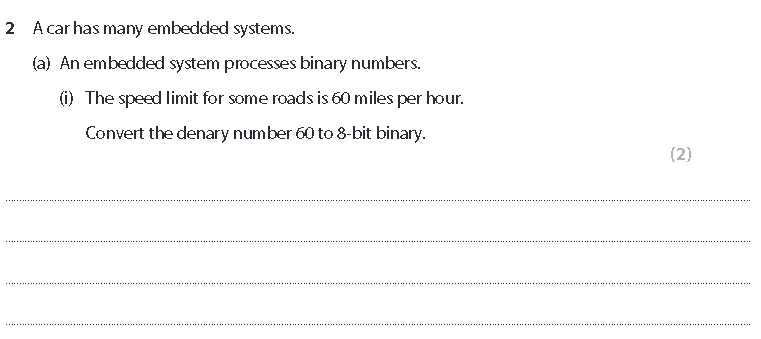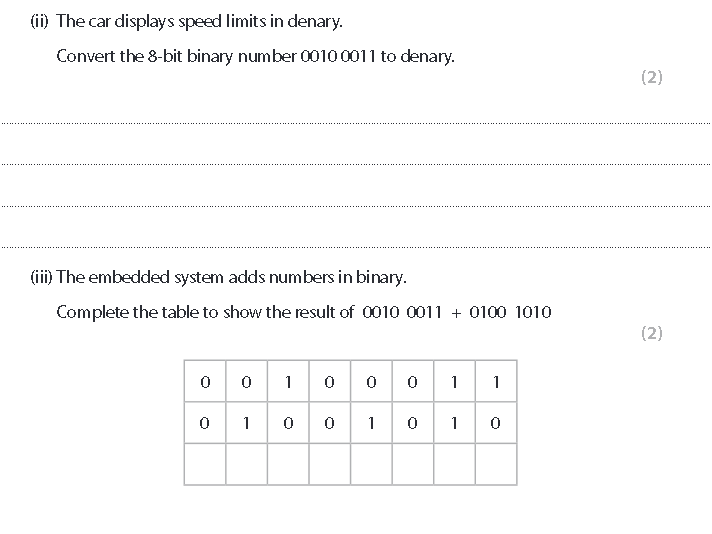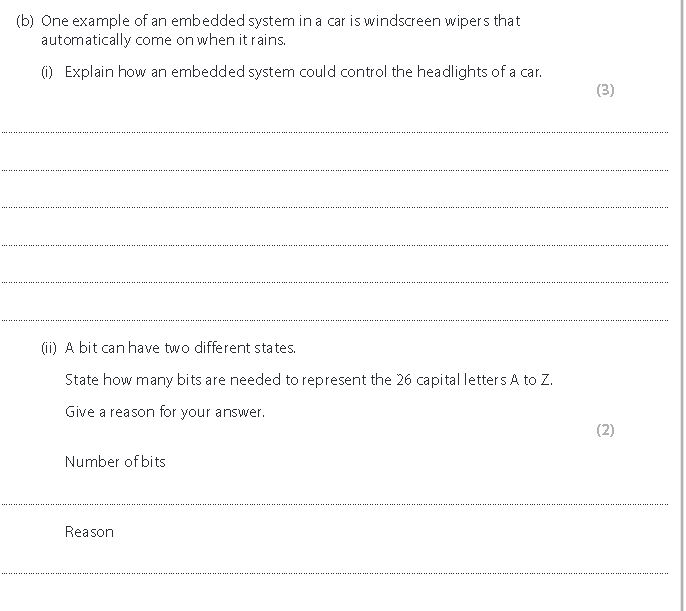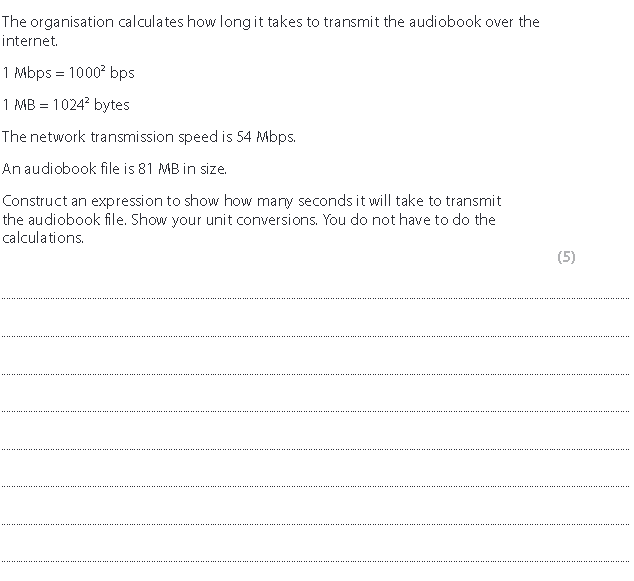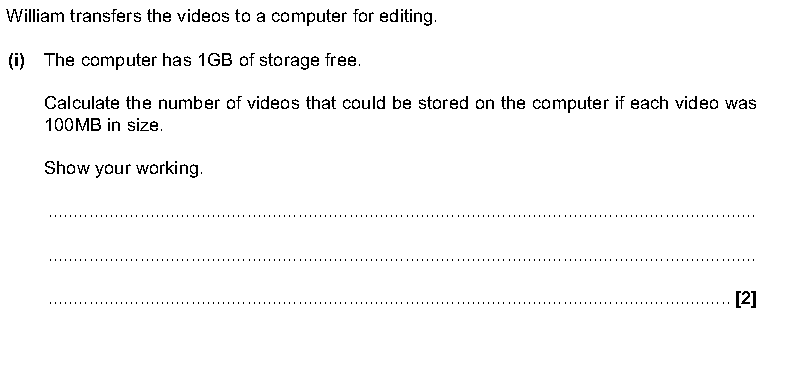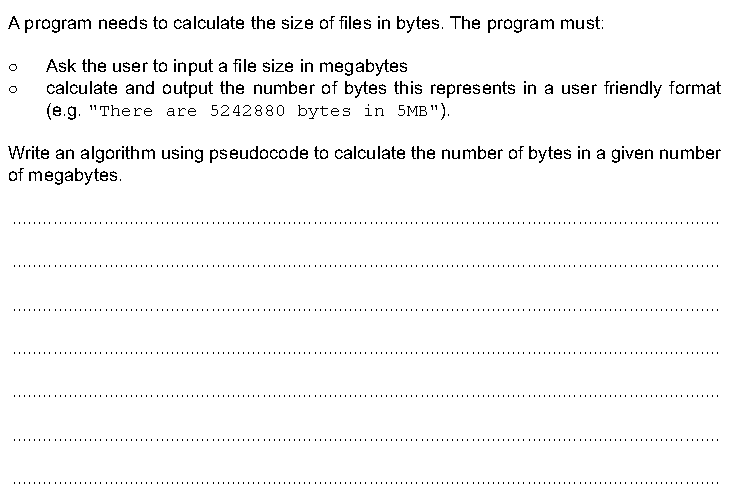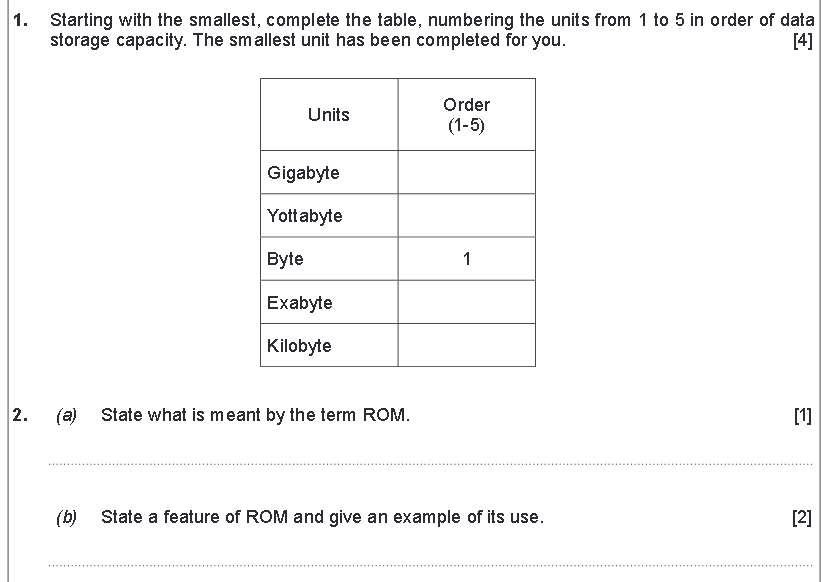Next: Create a python program to calculate bits and bytes in kilobytes and megabytes etc.

EXT: See if you can create a python program to create a binary clock.